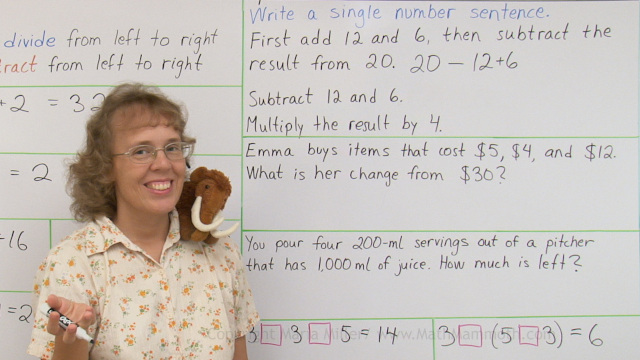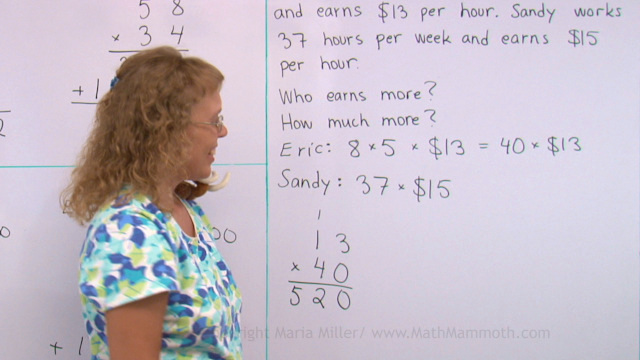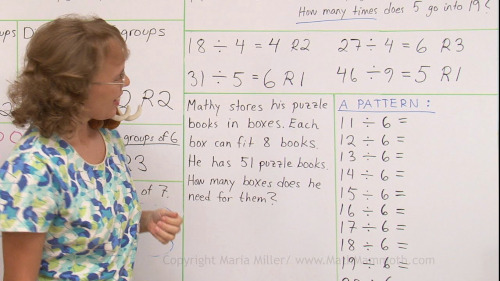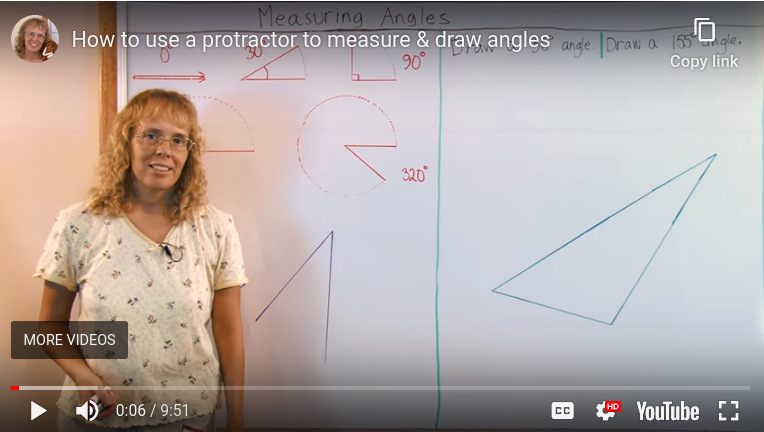^

# Free 4th grade math video lessons

This is a collection of free math videos for 4th grade, showing varied exercises for each topic. They match Math Mammoth Grade 4 curriculum but will also work no matter which curriculum you follow (in other words, the videos don't rely on you having Math Mammoth curriculum).

Please choose a topic from the list. Please realize this is not a complete coverage of all 4th grade math. There isn't (yet) a video for every lesson in Math Mammoth Grade 4 curriculum, and I hope to add to the collection in the future. Also, some of the videos are older work of mine, but listed here in case they are still helpful to some.

## Chapter 1: Addition, subtraction, rounding, and graphsBar models in addition and subtraction problems

The order of operations and writing simple expressions

Making a bar graph plus word problems

Rounding 4-digit numbers

Estimating the final result

Calculate and estimate money amounts

Calculate and estimate money amounts: word problems

## Chapter 2: Large Numbers and Place Value

Rounding and estimating with large numbers - up to six digits

Multiples of 10, 100, and 1000

## Chapter 3: Multi-Digit Multiplication"Scales" (pan balance) problems (2 videos)

Multiply in parts 1 (partial products) – 2-digit by 1-digit multiplication

Multiply in parts 2 – partial products algorithm for 3-digit numbers and money amounts

Estimating in multiplication: round the factors!

Multiplying in columns, the standard way — 1-digit by 2,3,4-digit multiplication; standard algorithm

The order of operations again

So many of the same thing

Multiply by whole tens in columns — aka a shortcut for 2-digit multiplication when one factor is a multiple of ten

The standard multiplication algorithm with a two-digit multiplier — aka two-digit multiplication, and why we add a zero on the 2nd line (2 videos)

## Chapter 4: Time and Measuring

Time units

Elapsed time to the minute

Measuring temperature — Celsius and Fahrenheit degrees

Measuring length (to the nearest 1/8 inch and in cm/mm)

Feed, Yards, and Miles (conversions between inches, feet, and yards)

Metric units for measuring length (from millimeter to kilometer)

Customary units of weight

Metric units of weight

Metric units of volume

## Chapter 5: Division and divisibilityThe order of operations plus writing simple expressions

The remainder, part 1

The remainder, part 2 – we learn to write division with a different symbol and learn about the remainder in word problems

How to teach long division

Long division — step-by-step examples (several videos)

Remainder problems – word problems involving long division with a remainder

Concept of average

Find fractional parts with division

Divisibility (and factors & multiples)

An introduction to prime numbers

Finding factors

## Chapter 6: GeometryVideos for 4th (and 5th) grade geometry are found on the same page. You can also use these shortcut links:

Review: Area versus perimeter

Lines, rays, and angles

Drawing angles (How to use the protractor)

Estimating angles

How to draw a right angle and a rectangle (for the lesson "Parallel and Perpendicular Lines"

Parallelograms

Triangles (acute, obtuse, and right)

## Chapter 7: Fractions

Mixed numbers and fractions

Adding mixed numbers (with like fractional parts)

Equivalent fractions

Subtracting fractions and mixed numbers (2 videos)

Multiplying fractions by whole numbers (2 videos)

## Chapter 8: Decimals

Decimals numbers — tenths

Two decimal digits — hundredths (2 videos)

Add and subtract decimals in columns (2 videos)

Add (and subtract) decimals mentally (2 videos)

Back to all videos index

WAIT!

Receive my monthly collection of math tips & resources directly in your inbox — and get a FREE Math Mammoth book!You can unsubscribe at any time.

### Math Mammoth Tour

Confused about the different options? Take a virtual email tour around Math Mammoth! You'll receive:

An initial email to download your GIFT of over 400 free worksheets and sample pages from my books. Six other "TOURSTOP" emails that explain the important things and commonly asked questions concerning Math Mammoth curriculum. (Find out the differences between all these different-colored series!)

This way, you'll have time to digest the information over one or two weeks, plus an opportunity to ask me personally about the curriculum.
A monthly collection of math teaching tips & Math Mammoth updates (unsubscribe any time)### "Mini" Math Teaching Course

This is a little "virtual" 2-week course, where you will receive emails on important topics on teaching math, including:

- How to help a student who is behind
- Troubles with word problems
- Teaching multiplication tables
- Why fractions are so difficult
- The value of mistakes
- Should you use timed tests
- And more!

A GIFT of over 400 free worksheets and sample pages from my books right in the very beginning.A monthly collection of math teaching tips & Math Mammoth updates (unsubscribe any time)
Enter your email to receive math teaching tips, resources, Math Mammoth news & sales, humor, and more! I tend to send out these tips about once monthly, near the beginning of the month, but occasionally you may hear from me twice per month (and sometimes less often).• A GIFT of over 400 free worksheets and sample pages from my books.